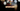# Leetcode | Solution of Valid Parentheses in JavaScript

March 30th, 2020
|

In this post, we will solve valid parentheses problem from leetcode and compute it's time & space complexity. Let's begin.

# Problem Statement

The question can be found at leetcode valid parentheses problem.

The problem states that we need to determine if a given string has all its parentheses balanced.

# Constraints and challenges

A string is valid if

• Open brackets must be closed by the same type of brackets.
• Open brackets must be closed in the correct order.

# Solution

We will discuss a solution using a stack and a hash map.

In this method, we will follow the following approach

• we will create a map of parentheses with opening parentheses as key and corresponding closing ones as value
• will declare an empty array to use as a stack• will check the string character by character
• if the character is an opening bracket will push it to stack
• if it is a closing bracket, will check the last entry in the stack
• if the last entry is an opening bracket of the same type, will pop that from the stack
• else, the string is not balanced
• at last, when we have checked all the characters, the stack should be empty, why?• to keep the string balanced, each closing bracket will have an opening one, and since when this case occurs, we clear the last entry from stack. Hence the stack should be empty after the iteration to ensure the string is balanced.

We have discussed the approach, I urge you to go ahead on leetcode and give it another try.If you are here, it means something went wrong in implementation or you are just too lazy. In any case, let's see a simple implementation of the above logic.
``````var isValid = function (s) {

const obj = {
"(": ")",
"{": "}",
"[": "]",
}

const stack = [];

for (const paran of s) {
if (obj.hasOwnProperty(paran)) {
stack.push(paran)
} else {
const closeParan = stack.pop();
if (paran !== obj[closeParan]) {
return false;
}
}
}

return stack.length === 0;
};``````

Let's discuss the implementation.

We declare a hashmap with opening brackets as key and closing ones as their values. Next, we initialize an empty array and will use it as our stack to store opening braces.

Next, we are looping over all the characters of the string, and in this case, all the braces present in the string. In the next condition, we check if the character is an opening bracket, if yes we push it to the stack.

If the character is a closing bracket, we check for the last entry in the stack. If the last entry in the stack is the opening counterpart of the current closing bracket, we remove the entry from stack. It balances the bracket. If no, the braces are not balanced, hence we return false and exit.

In the end, if the stack is not empty, it means there was some opening bracket for which closing bracket was not found in order. Thus we return false or true depending on stack length.

This is all we need to solve the problem, once we submit it, these are the stats.

``````Status: Accepted
Runtime: 56ms
Memory: 33MB``````

## Time and space complexity

### Time complexity

We loop over the characters of the string for every string. We also use some of the methods on object and stack, but they are constant time, so time complexity would be O(len S), proportional to the length of the string.

### Space complexity

We are taking a string as input, but also using a stack to keep characters of the string. In the worst case (no closing bracket in the string ), we will end up storing all the characters in the stack. We have an object but the size is always constant. So space will again be proportional to the length of the string. O(len S).

# Summary

So, we solved the valid parentheses problem, used a stack and a hashmap and saw a couple of object method. At last, calculated the time and space complexities.

I hope you enjoyed solving this question. This is it for this one, complete source code for this post can be found on my Github Repo. Will see you in the next one.